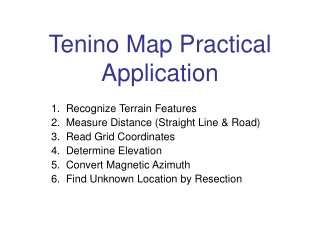DownloadDownload PresentationTenino Map Practical Application

# Tenino Map Practical Application

Télécharger la présentation## Tenino Map Practical Application

- - - - - - - - - - - - - - - - - - - - - - - - - - - E N D - - - - - - - - - - - - - - - - - - - - - - - - - - -
##### Presentation Transcript

1. Tenino Map Practical Application 1. Recognize Terrain Features 2. Measure Distance (Straight Line & Road) 3. Read Grid Coordinates 4. Determine Elevation 5. Convert Magnetic Azimuth 6. Find Unknown Location by Resection

2. Note Grid Lines (Read Right and up) Read up = 78 Note Meter Scale Note Miles Scale Read Right = 15 Note Contour Interval Note G-M Angle Back Azimuth = Add or Subtract 180 Note Map Number

3. Practical Exercise #11. Recognize Terrain Feature (Hill Top) 2. Identify Grid Square • Look at the lower left hand corner of your map, and locate Hill Top 192. • What grid square is Hill Top 192 located in?

5. Practical Exercise # 2Find Eight-Digit Grid Coordinates • What are the Eight Digit Grid Coordinates for Hill Top 192?

6. Answer • How many of you came up with EG 02457910 ?

7. Practical Exercise #3Identify Terrain Feature • What natural terrain feature is at grid coordinate EG06109015?

9. Practical Exercise #4 Identify Terrain Feature • Locate north/south grid line 15 at the bottom of your map. • Follow it up six grid squares. • What man-made Terrain Feature do you see in this grid square? (Remember: Read Right & Up)

10. Answer • How many have “Mine or Quarry”?

11. Practical Exercise #5Determine Eight-Digit Grid Coordinates • What are the eight-digit grid coordinates of the mine or quarry you have just located?

12. Answer • How many have EG15818215?

13. Measure Straight-line Distance Match tick marks on paper strip against map scale.

14. Practical Exercise #6Measure Straight-line Distance in Meters • What is the straight-line distance in meters between the water tower in grid square EG11558558 and the oil tank in grid square EG08359360?

15. Answer • How many have 8,630 meters? (+/- 100 Meters)

16. Practical Exercise #7Measure Straight Line Distance in Miles • What is the distance in miles between those same two points? (Use “Statute” Miles Scale, not “Nautical” Miles Scale)

17. Answer • 5.4 Statute Miles (+/- .10 miles)

18. Measuring Curved Line Distance(As with Roads) Move and change angle of paper strip, as you move along road.

19. Practical Exercise #8Measure Road Distance • What is the road distance in meters between the river bridge in grid square EG10408840, southwest to the road junction in grid square EG03108525,at the Church of God?

20. Answer • 8,000 meters (+/- 100 meters)

21. Determine Elevation Locate point on the map Determine contour interval of map Locate index contour line nearest point Count number of contour lines up or down that must be crossed to get to point Points at top of hill add half the contour interval Points at bottom of depression subtract half the contour interval

22. Practical Exercise #91. Recognize Natural Terrain Feature2.Determine Elevation • What type of natural terrain feature is at grid coordinate EG16107925? (Look closely, before identifying) • What is its height in meters?

23. Answers • Saddle • 150 meters (+/- 10 meters) (Did you remember what the contour interval is on the Tenino Map?)

24. Practical Exercise #101. Recognize a Natural Terrain Feature2. Determine Elevation • What natural feature is found at grid coordinate EG17078440 • What is its elevation in meters?

25. Answers • Hill Top • 310 Meters

26. Convert Magnetic Azimuth Check Here on Your Tenino Map for GM Angle Instructions

27. Practical Exercise #111. Convert Magnetic Azimuth 2. Determine Eight-Digit Grid Coordinates3. Measure Straight line distance4. Recognize Manmade Feature • An observer at the Crawford Mountain Lookout Tower, in grid square EG1887 sees a convoy parked on a light duty road. The magnetic azimuth from his position to the convoy is 260 degrees, a distance of 15,820 meters. What is the eight-digit coordinate of the convoy.

28. Answer • EG02509065 (+/- 50 meters) (+50 meters = EG02559070) ( -50 meters = EG02459060)

29. Practical Exercise #121.Convert Magnetic Azimuth 2. Find Unknown Location. • You are at the church of God in grid square EG0385 and observe an automobile accident on Interstate 5. The magnetic azimuth from your position to the cars is 331 degrees. What is the eight-digit coordinate of the accident?

30. Answer • EG02329010 (+/- 50 meters) (+ 50 meters = EG23379015) (- 50 meters = EG02279005)

31. Determine Unknown Location on Ground by Resection Step 1: Identify Two Or Three Distant Locations on the Ground and Mark them on the Map Step 2: Shoot a Magnetic Azimuth to the Distant Locations Step 3: Convert the Magnetic Azimuths to Grid Azimuths Step 4: Compute the Back Azimuths Remember: a. If Converted Grid Azimuth is less than 180 , add 180 . b. If Converted Grid Azimuth is more than 180, subtract 180

32. Practical Exercise #131.Locate Unknown Point on Ground by Resection • You are trying to determine your unknown location. You determine the magnetic azimuth to TV relay tower in grid square EG1287 to be 204 degrees and the magnetic azimuth to Crawford Mountain Lookout Tower in grid square EG1887 to be 129 degrees. What is your location to the nearest 50 meters?

33. Answer • EG15859120 (+/- 50 meters) + 50 meters = EG15909125 - 50 meters = EG15809115 (Did you remember to first add the G-M Angle, and then subtract or add 180 degrees?)

34. Practical Exercise #141. Identify Manmade Feature 2. Convert Magnetic Azimuth 3. Measure Straight Line Distance 4. Determine Grid Coordinates • What manmade feature is on a magnetic azimuth of 119 degrees at a distance of 5,700 meters from bench mark 86 in grid square EG0589?